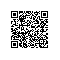# 【C#】2.算法温故而知新 - 冒泡排序using System;
using System.Collections.Generic;
using System.Linq;
using System.Text;

namespace ConsoleApplication1
{
public class Program
{
public static void Main(string[] args)
{
int[] nums = new int { 20, 14, 9, 22, 18, 0, 1, 8, 29, 28 };//初始化一个数组,其中有10个数，这里假定是我们输入的数，需要从小到大排序
int temp;
//冒泡排序核心部分
for (int i = 1; i <= nums.Length - 1; i++)//n个数排序。只用进行n-1趟交换
{
for (int j = 1; j <= nums.Length - i; j++)//从第一位开始比较知道最后一个尚未归位的数，想一下为什么到n-1就可以了
{
if (nums[j - 1] > nums[j])//比较大小并交换
{
temp = nums[j - 1];
nums[j - 1] = nums[j];
nums[j] = temp;
}
}
}

for (int i = 0; i < nums.Length; i++)
Console.Write("  " + nums[i]);
}
}
}使用钉钉扫一扫加入圈子
+ 订阅